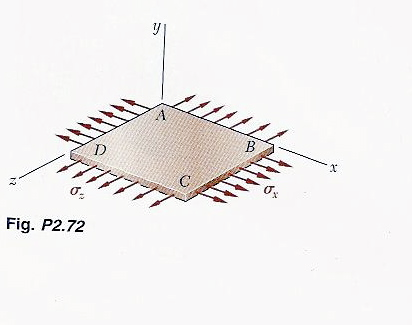# The homogeneous plate ABCD is subjected to a biaxial loading asshown. It is known that \sigma_z=\sigma_o and that the change in length of the plate ine1s2kat26 2021-03-28 AnsweredThe homogeneous plate ABCD is subjected to a biaxial loading asshown. It is known that ${\sigma }_{z}={\sigma }_{o}$ and that the change in length of the plate in the x direction must be zero, that is ${ϵ}_{x}=0$. Denoting E the modulus of elasticity and by v Poisson's ratio, determine (a) the required magnitude of ${\sigma }_{x}$, and (b) the ratio $\frac{{\sigma }_{o}}{{ϵ}_{z}}$
You can still ask an expert for help

• Questions are typically answered in as fast as 30 minutes

Solve your problem for the price of one coffee

• Math expert for every subject
• Pay only if we can solve itgotovub
a) Strain in x-direction, ${ϵ}_{x}=\frac{{\sigma }_{x}}{E}-V\frac{{\sigma }_{z}}{E}$
But given, ${ϵ}_{x}=0,{\sigma }_{z}={\sigma }_{o}$
$\frac{{ϵ}_{x}}{E}-V\frac{{\sigma }_{o}}{E}=0$
Then find ${\sigma }_{x}$
b) Strain in Z-direction, ${ϵ}_{Z}=\frac{{\sigma }_{Z}}{E}-V\frac{{\sigma }_{x}}{E}$
${ϵ}_{Z}=\frac{{\sigma }_{0}}{E}-V\frac{{\sigma }_{x}}{E}$
Then find $\frac{{\sigma }_{0}}{{ϵ}_{z}}$